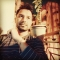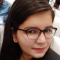## Difference between PHP and CUpdated on 10-Aug-2022 07:16:55
Many developers will indeed agree that comparing C Programming and PHP is unfair because they differ in web development. PHP is by far the most famous serverside scripting language. JavaScript works with things on the client end without ever returning to the server, while PHP manages things on the application server. PHP is based on the C programming language, thus everyone with a basic understanding of C will find it easy to learn PHP. What is PHP? PHP is a general−purpose programming language that is primarily utilized in the development of websites. It was developed in 1994 by DanishCanadian programmer ... Read More

## fopen() for existing file in write mode in CUpdated on 01-Feb-2022 08:35:23
fopen() method in C is used to open the specified file.Let’s take an example to understand the problemSyntaxFILE *fopen(filename, mode)The following are valid modes of opening a file using fopen(): ‘r’, ‘w’, ‘a’, ‘r+’, ‘w+’, ‘a+’. For details visit visit C library function - fopen()fopen() for an existing file in write modeIf the file to be opened does not exist in the current directory then a new empty file with write mode is created.If the file to be opened exists in the current directory and is open using ‘w’ / ‘w+’, the contents will be deleted before writing.ExampleProgram to illustrate ... Read More

## C Program to Redeclaration of global variableUpdated on 03-Nov-2021 05:22:57
We will understand how C and C++ behave differently in case we re-declare a global variable without initializing, redeclaring global variables with initialization, redeclaring global variables and initializing them twice. Also, we will repeat above combinations with local variables.1. A) C program : Redeclaring Global variables with no initialization#include int var; int var; int main(){    printf("Var = %d",var);    return 0; }OutputVar = 0B) C++ program : Redeclaring Global variables with no initialization#include using namespace std; int var; int var; int main(){    cout

## C Program for Recursive Bubble SortUpdated on 02-Nov-2021 07:58:22
Bubble Sort is one of the simplest sorting algorithms used to sort data by comparing the adjacent elements. All the elements are compared in phases. The first phase places the largest value at the end, the second phase places the second largest element at the second last position and so on till the complete list is sorted.Bubble Sort Algorithmint arr= { 5, 4, 2, 1, 3 };int i, j ;Traverse from index i=0 to iarr[j] swap arr[i] with arr[j]EndRecursive Bubble SortIf array length is 1 then returnTraverse array once and fix largest element at the endRecursively perform step 2 for ... Read More

## C program to demonstrate usage of variable-length arraysUpdated on 11-Oct-2021 11:52:27
Suppose we are in charge of building a library system that monitors and queries various operations at the library. We are now asked to implement three different commands that perform the following −By using command 1, we can record the insertion of a book with y pages at shelf x.By using command 2, we can print the page number of the y-th book at shelf x.By using command 3, we can print the number of books on shelf x.The commands are given to us as a 2D array in this format {command type, x, y}. If there is no y ... Read More

## C program to find out the maximum value of AND, OR, and XOR operations that are less than a given valueUpdated on 11-Oct-2021 11:36:58
Suppose we are given two integers k and n. Our task is to perform three operations; bitwise AND, bitwise OR, and bitwise XOR between all pairs of numbers up to range n. We return the maximum value of all three operations between any two pairs of numbers that is less than the given value k.So, if the input is like n = 5, k = 5, then the output will be 4 3 4.The greatest value of AND, OR, and XOR operations between all pairs of numbers that are less than 5 are 4, 3, and 4 respectively. We can ... Read More

## C program to sort triangles based on areaUpdated on 08-Oct-2021 11:26:27
Suppose we have an array of different triangles where triangles[i] = [ai, bi, ci] these are the sides of ith triangle. We shall have to sort the triangles based on their area. The area of a triangle by using sides is: square root of p*(p-a)*(p-b)*(p-c) where p = (a+b+c)/2.So, if the input is like (7, 24, 25), (5, 12, 13), (3, 4, 5), then the output will be (3, 4, 5), (5, 12, 13), (7, 24, 25)To solve this, we will follow these steps −Define triangle object with sides a, b and cDefine a function square(), this will take Triangle ... Read More

## C program to find sum, max and min with Variadic functionsUpdated on 08-Oct-2021 11:23:16
Suppose we want to make some functions that can take multiple arguments, there are no fixed number of arguments. We want to make three functions sum(), max() and min(), they can calculate sum of the numbers, maximum of numbers and minimum of given numbers respectively. Each of these functions will take number of arguments count as their first argument. To define this type of functions we need to use ellipsis (...) three dots into the function argument. To use it we shall have to include stdarg.h header file. This type of function is called variadict functions. To access variable arguments ... Read More

## C program to find permutations of given stringsUpdated on 08-Oct-2021 11:19:50
Suppose we have few strings in an array. We shall have to find all permutations of them in different line.So, if the input is like strings = ["abc", "def", "ghi"], then the output will beabc def ghi abc ghi def def abc ghi def ghi abc ghi abc def ghi def abcTo solve this, we will follow these steps −Define a function next_permutation(), this will take n, string array s, for initialize i := n - 1, when i > 0, update (decrease i by 1), do:if s[i] > s[i - 1]), then:j := i + 1for j < n, ... Read More

## C program to find frequency of each digit in a stringUpdated on 08-Oct-2021 11:18:19
Suppose we have a string s. The s contains letters and digits both. We shall have to find frequencies of each digit and display them. To do this we can make an array of size 10 for each digits (0 through 9), initially all elements are 0 inside the array, then when we encounter a digit simply increase the value of that index and finally print them all.So, if the input is like s = "we85abc586wow236h69", then the output will be (Number 2, Freq 1) (Number 3, Freq 1) (Number 5, Freq 2) (Number 6, Freq 3) (Number 8, Freq ... Read More